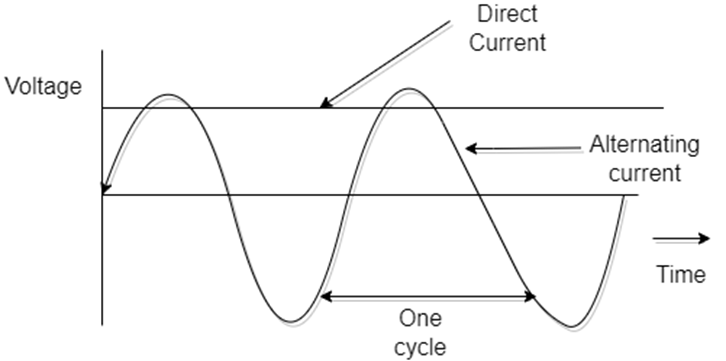# Difference between active power, reactive power, and apparent power## What is meant by Electrical power?

The rate at which the work is being done in an electrical circuit is called an electrical power.

Electrical power is defined as the rate of transferred energy. Unit of power in watts. Power can be generated by a generator or Electrical Batteries, the consumed energy can be converted to other forms of energy like heat, motion, etc.

Electric power is of two types

1. AC Power

2. DC Power

Electrical Power= work done by an electrical current/Time

P=Vit/t= VI=IR² =V²/R

Where, V=Voltage in volts,

I= Current in Amperes,

R= Resistance

T= Time in seconds             P=power in watts

### What is meant by DC power?

DC stands for “Direct Current” is the one-directional flow of electric charge, Direct current may flow through semiconductors, Insulators, or Vacuum, The electric current flows in a constant direction

Uses

DC current is mainly used to charge the batteries, DC current is used in smelting Aluminum. It is also used in railways.

### What is AC power?

AC stands for ‘Alternating Current‘ which means the current alternatingly changes direction. This AC current is better for transporting current over long distances, which is why we use it for electricity transmission. For Domestic and Industrial (commercial), and is also present when audio and radio signals are carried on electrical wires

AC is used for transporting electric power through the electric grid from generators to end-users.

### Why DC power supply has a Power Factor?

In DC, the response of Capacitor and Inductor, Resistance.

Resistance:

The purely Resistance in DC circuit, the angle between the voltage and the current is 0˚, The power factor for a Resistance circuit is

PF=cos 0˚

Cos 0˚=1

P.F.= 1 (Unity).

Capacitor:

It’s the current that flows through the capacitor, C is the capacitance of the capacitor and

The voltage across capacitor is dv/dt

Current I=C *dv/dt

In DC the rate of change of voltage is zero, Current leads the voltage by 90˚

Cos 90˚=0,

So dv/dt =0,Since the DC doesn’t change its voltage so I= C *0, I=0.

The current (I)through a capacitor (C) is zero, so in DC source capacitor acts as an open circuit, therefore the open circuit doesn’t have a power factor.

Inductor:

Current (I) flows through an inductor circuit is DC, then voltage V across the inductor is

V=L*di/dt

di/dt=Change of current

Change of current in DC is zero, so di/dt=0, Current lags the voltage by 90˚

Cos 90˚=0

Voltage through the inductor is zero, the voltage across any component is zero which means Resistance is zero, The Inductor act as a short circuit.

So, Capacitor- open circuit & Inductor -Short Circuit Remaining Resistance-power factor is one.

So, It concludes that DC power neglects the power factor in the circuit.

### What is the Power factor?

Power factor is defined as the cosine of the angle between voltage and current

Power Factor = Cosθ= kW/kVA = True power/Apparent power

### What are the types of power in an AC circuit?

1. Apparent power, Denoted by the symbol (S)

2. Active power, Denoted by the symbol (P)

3. Reactive power, Denoted by the symbol (Q)

### What is apparent power?

It is the product of the RMS value of the applied voltage and current, It is known as wattless or idle power.

Apparent power    S=V*I

### What is active Power?

It is the power that is developed in the circuit resistance

It is also known as True power

P=I²R, P = E²/R

Active power, P =V*I* Cosθ.

In an AC circuit, active power is consumed by the equipment to do useful work

### What is reactive power?

It is the power that developed in the circuit reactance.   It is an unwanted part of the energy supplied, A minimum value of reactive power is always required to keep the voltage constant and supply useful Active power

Q=I²X, P =E²/X

Active power, P =V*I* sinθ.

### What is the difference between Active Power and Apparent Power?

Apparent power is a combination of Real power and Reactive power, True power is a result of resistive components and reactive power is a result of capacitive and inductive components, these components are implemented commonly in all circuits.

### 1. What is power?

Power is the rate of the work done and its unit is joule/second or watt

It can be defined as one coulomb of electric charges moving through a potential difference of one volt in one second, the rate of work is one joule per second or one watt. hence power is obtained as a product of the voltage and current in the Electric Circuit.

P= E*I,P=I²R=E²R      Where E=Voltage,I=current.

### 2. What is horsepower?

Horsepower (hp) is a unit of power, the work of a horse is done at a rate of time, with reference to the output of engines or motors. The horsepower was invented by James Watt. Horse power refers to the power an engine produces.

1hp=745.7watts

hp= Fd/t

### 3. What is the reason for the DC power supply is not being used in transmission?

DC is not used for transmission because DC goes heavy attenuation while transmission over long distance as we don’t transform it from low voltage.

### 4. What is Phasor?

Phasor is nothing but a vector that is rotating at a constant angular velocity. The length of the phasor or vector represents the magnitude of alternating quantity and the arrow indicates the direction of the vector.

Pure Resistance

Pure Capacitance

Pure Inductance

Scroll to Top